# Wavelet Denoising¶

This numerical tour uses wavelets to perform non-linear image denoising. $\newcommand{\dotp}{\langle #1, #2 \rangle}$ $\newcommand{\qandq}{\quad\text{and}\quad}$ $\newcommand{\qwhereq}{\quad\text{where}\quad}$ $\newcommand{\qifq}{ \quad \text{if} \quad }$ $\newcommand{\ZZ}{\mathbb{Z}}$ $\newcommand{\RR}{\mathbb{R}}$ $\newcommand{\pa}{\left(#1\right)}$ $\newcommand{\si}{\sigma}$ $\newcommand{\Nn}{\mathcal{N}}$ $\newcommand{\Bb}{\mathcal{B}}$ $\newcommand{\EE}{\mathbb{E}}$ $\newcommand{\norm}{\|#1\|}$ $\newcommand{\abs}{\left|#1\right|}$ $\newcommand{\choice}{ \left\{ \begin{array}{l} #1 \end{array} \right. }$ $\newcommand{\al}{\alpha}$ $\newcommand{\la}{\lambda}$ $\newcommand{\ga}{\gamma}$ $\newcommand{\Ga}{\Gamma}$ $\newcommand{\La}{\Lambda}$ $\newcommand{\si}{\sigma}$ $\newcommand{\Si}{\Sigma}$ $\newcommand{\be}{\beta}$ $\newcommand{\de}{\delta}$ $\newcommand{\De}{\Delta}$ $\newcommand{\phi}{\varphi}$ $\newcommand{\th}{\theta}$ $\newcommand{\om}{\omega}$ $\newcommand{\Om}{\Omega}$

In :
using PyPlot
using NtToolBox
arequire("NtToolBox")

WARNING: Base.UTF8String is deprecated, use String instead.
WARNING: Base.UTF8String is deprecated, use String instead.
WARNING: Base.UTF8String is deprecated, use String instead.
WARNING: Base.UTF8String is deprecated, use String instead.
WARNING: Base.UTF8String is deprecated, use String instead.
WARNING: Base.UTF8String is deprecated, use String instead.
likely near In:4
WARNING: Base.UTF8String is deprecated, use String instead.
likely near In:4
WARNING: Base.UTF8String is deprecated, use String instead.
likely near In:4
WARNING: Base.UTF8String is deprecated, use String instead.
likely near In:4
WARNING: Base.UTF8String is deprecated, use String instead.
likely near In:4
WARNING: Base.UTF8String is deprecated, use String instead.
likely near In:4
WARNING: Base.UTF8String is deprecated, use String instead.
likely near In:4
WARNING: Base.UTF8String is deprecated, use String instead.
likely near In:4
WARNING: Base.UTF8String is deprecated, use String instead.
likely near In:4
WARNING: Base.UTF8String is deprecated, use String instead.
likely near In:4
WARNING: Base.UTF8String is deprecated, use String instead.
likely near In:4
WARNING: Base.UTF8String is deprecated, use String instead.
likely near In:4
WARNING: Base.UTF8String is deprecated, use String instead.
likely near In:4
WARNING: Base.UTF8String is deprecated, use String instead.
likely near In:4
WARNING: Base.UTF8String is deprecated, use String instead.
likely near In:4
WARNING: Base.UTF8String is deprecated, use String instead.
likely near In:4
WARNING: Base.UTF8String is deprecated, use String instead.
likely near In:4
WARNING: Base.UTF8String is deprecated, use String instead.
likely near In:4
WARNING: Base.UTF8String is deprecated, use String instead.
likely near In:4
WARNING: Base.UTF8String is deprecated, use String instead.
likely near In:4
WARNING: Base.UTF8String is deprecated, use String instead.
likely near In:4
WARNING: Base.UTF8String is deprecated, use String instead.
likely near In:4
WARNING: Base.UTF8String is deprecated, use String instead.
likely near In:4
WARNING: Base.UTF8String is deprecated, use String instead.
likely near In:4
WARNING: Base.UTF8String is deprecated, use String instead.
likely near In:4
WARNING: Base.UTF8String is deprecated, use String instead.
likely near In:4
WARNING: Base.UTF8String is deprecated, use String instead.
likely near In:4
WARNING: Base.UTF8String is deprecated, use String instead.
likely near In:4
WARNING: Base.UTF8String is deprecated, use String instead.
likely near In:4
WARNING: Base.UTF8String is deprecated, use String instead.
likely near In:4
WARNING: Base.UTF8String is deprecated, use String instead.
likely near In:4
WARNING: Base.UTF8String is deprecated, use String instead.
likely near In:4
WARNING: Base.UTF8String is deprecated, use String instead.
likely near In:4
WARNING: Base.UTF8String is deprecated, use String instead.
likely near In:4
WARNING: Base.UTF8String is deprecated, use String instead.
likely near In:4
WARNING: Base.UTF8String is deprecated, use String instead.
likely near In:4
WARNING: Base.UTF8String is deprecated, use String instead.
likely near In:4
WARNING: Base.UTF8String is deprecated, use String instead.
likely near In:4
WARNING: Base.UTF8String is deprecated, use String instead.
likely near In:4
WARNING: Base.UTF8String is deprecated, use String instead.
likely near In:4
in depwarn(::String, ::Symbol) at .\deprecated.jl:64
in #parse_file#6(::Array{Any,1}, ::Function, ::String) at C:\Users\Ayman\.julia\v0.5\Autoreload\src\files.jl:63
in execute_request(::ZMQ.Socket, ::IJulia.Msg) at C:\Users\Ayman\.julia\v0.5\IJulia\src\execute_request.jl:157
in eventloop(::ZMQ.Socket) at C:\Users\Ayman\.julia\v0.5\IJulia\src\eventloop.jl:8
WARNING: replacing module NtToolBox


## Image Denoising¶

We consider a simple generative model of noisy images $F = f_0+W$ where $f_0 \in \RR^N$ is a deterministic image of $N$ pixels, and $W$ is a Gaussian white noise distributed according to $\Nn(0,\si^2 \text{Id}_N)$, where $\si^2$ is the variance of noise.

The goal of denoising is to define an estimator $\tilde F$ of $f_0$ that depends only on $F$, i.e. $\tilde F = \phi(F)$ where $\phi : \RR^N \rightarrow \RR^N$ is a potentially non-linear mapping.

Note that while $f_0$ is a deterministic image, both $F$ and $\tilde F$ are random variables (hence the capital letters).

The goal of denoising is to reduce as much as possible the denoising error given some prior knowledge on the (unknown) image $f_0$. A mathematical way to measure this error is to bound the quadratic risk $\EE_w(\norm{\tilde F - f_0}^2)$, where the expectation is computed with respect to the distribution of the noise $W$.

Image loading and adding Gaussian Noise For real life applications, one does not have access to the underlying image $f_0$. In this tour, we however assume that $f_0$ is known, and $f = f_0 + w\in \RR^N$ is generated using a single realization of the noise $w$ that is drawn from $W$. We define the estimated deterministic image as $\tilde f = \phi(f)$ which is a realization of the random vector $\tilde F$.

First we load an image $f_0 \in \RR^N$ where $N=n \times n$ is the number of pixels, and then display it.

In :
n = 256
name = "NtToolBox/src/data/flowers.png"
imageplot(f0, "Original image")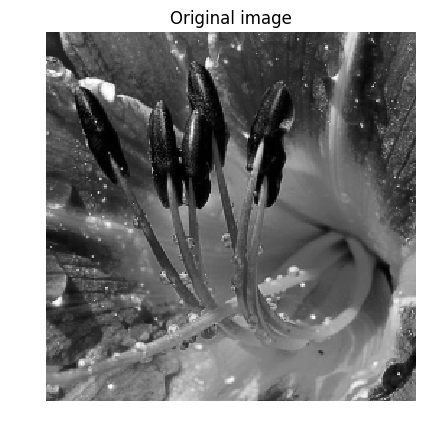WARNING: Base.UTF8String is deprecated, use String instead.
likely near C:\Users\Ayman\.julia\v0.5\IJulia\src\kernel.jl:31
WARNING: Base.UTF8String is deprecated, use String instead.
likely near C:\Users\Ayman\.julia\v0.5\IJulia\src\kernel.jl:31

Out:
PyObject <matplotlib.text.Text object at 0x000000001E560BE0>
WARNING: Base.UTF8String is deprecated, use String instead.
likely near C:\Users\Ayman\.julia\v0.5\IJulia\src\kernel.jl:31
WARNING: Base.UTF8String is deprecated, use String instead.
likely near C:\Users\Ayman\.julia\v0.5\IJulia\src\kernel.jl:31
WARNING: Base.UTF8String is deprecated, use String instead.
likely near C:\Users\Ayman\.julia\v0.5\IJulia\src\kernel.jl:31
WARNING: Base.UTF8String is deprecated, use String instead.
likely near C:\Users\Ayman\.julia\v0.5\IJulia\src\kernel.jl:31


Standard deviation $\si$ of the noise.

In :
sigma = .08;

Out:
0.08

Then we add Gaussian noise $w$ to obtain $f=f_0+w$.

In :
using Distributions
f = f0 + sigma.*rand(Normal(), size(f0), size(f0));

Out:
256×256 Array{Float64,2}:
0.197767  -0.0257282  -0.0123759  …  0.155727  0.0529168  0.325971
0.145914   0.13758     0.224535      0.400507  0.294812   0.476433
0.368774   0.155882    0.162269      0.426328  0.472055   0.437452
0.179438   0.361938    0.176758      0.213828  0.463064   0.495666
0.374562   0.104094    0.0776449     0.494927  0.460137   0.339856
0.250857   0.202362    0.351659   …  0.357698  0.451573   0.50062
0.301836   0.134737    0.122904      0.34439   0.286502   0.555476
0.294692   0.165099    0.335817      0.445586  0.485572   0.498635
0.138957   0.174414    0.188458      0.326686  0.383657   0.391858
0.224599   0.255533    0.0750932     0.282518  0.230207   0.357829
0.238367   0.177058    0.0318645  …  0.355176  0.446526   0.214903
0.151098   0.0564628   0.0627512     0.370991  0.405262   0.295054
0.260319   0.20488     0.199456      0.389186  0.307049   0.223367
⋮                                 ⋱                       ⋮
0.331763   0.618939    0.63287       0.484378  0.415202   0.469769
0.418583   0.680169    0.730723   …  0.408707  0.456117   0.548158
0.478348   0.590665    0.511442      0.433922  0.406942   0.435246
0.650441   0.758906    0.815282      0.486954  0.593717   0.488212
0.490201   0.594615    0.528997      0.530642  0.445585   0.505978
0.517794   0.714222    0.576748      0.541272  0.43274    0.457139
0.584285   0.531977    0.512877   …  0.340489  0.530489   0.526308
0.59392    0.587088    0.268857      0.550497  0.548882   0.692324
0.406723   0.467705    0.35933       0.519929  0.484253   0.580409
0.484997   0.245728    0.343869      0.51918   0.452523   0.465082
0.364785   0.297215    0.385938      0.48377   0.394288   0.502843
0.172342   0.208223    0.316236   …  0.315482  0.364748   0.535831

Display the noisy image.

In :
imageplot(clamP(f), string("Noisy, SNR = ", string(snr(f0,f)) ))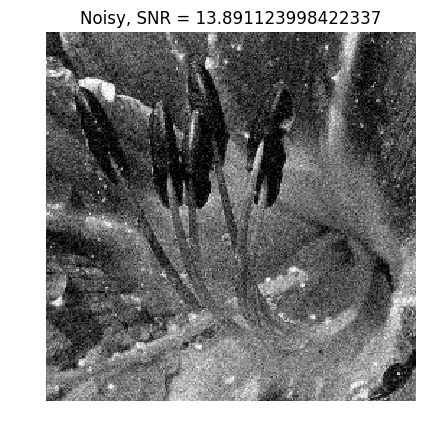Out:
PyObject <matplotlib.text.Text object at 0x000000001EA53BE0>

## Hard Thresholding in Wavelet Bases¶

A simple but efficient non-linear denoising estimator is obtained by thresholding the coefficients of $f$ in a well chosen orthogonal basis $\Bb = \{\psi_m\}_m$ of $\RR^N$.

In the following, we will focuss on a wavelet basis, which is efficient to denoise piecewise regular images.

The hard thresholding operator with threshold $T \geq 0$ applied to some image $f$ is defined as $$S_T^0(f) = \sum_{\abs{\dotp{f}{\psi_m}}>T} \dotp{f}{\psi_m} \psi_m = \sum_m s_T^0(\dotp{f}{\psi_m}) \psi_m$$ where the hard thresholding operator is $$s_T^0(\alpha) = \choice{ \alpha \qifq \abs{\al}>T, \\ 0 \quad\text{otherwise}. }$$

The denoising estimator is then defined as $$\tilde f = S_T^0(f).$$

Display the function $s_T^0(\al)$ for $T=1$.

In :
thresh_hard = (u, t) -> u.*(abs(u) .> t)
alpha = linspace(-3, 3, 1000)
plot(alpha, thresh_hard(alpha, 1))
axis("equal")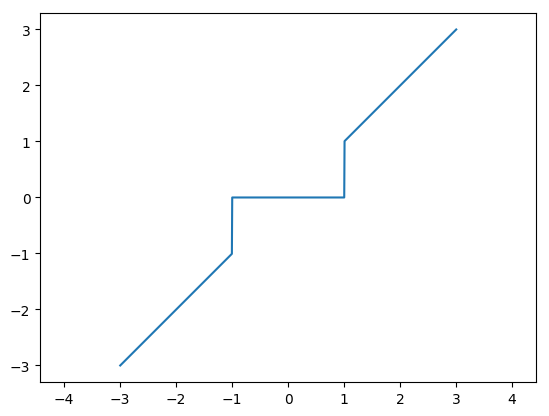Out:
(-3.3,3.3,-3.3,3.3)

Parameters for the orthogonal wavelet transform.

In :
h = [0, .482962913145, .836516303738, .224143868042, -.129409522551]
h = h./norm(h)
Jmin = 2;

Out:
2

First we compute the wavelet coefficients $a$
of the noisy image $f$.

In :
a = perform_wavortho_transf(f, Jmin, +1, h);

Out:
256×256 Array{Float64,2}:
23.4035      23.9434      18.6651     20.8802     …  -0.0239512  -0.00379351
13.6463      11.6067      18.1772     21.5199        -0.0969946  -0.108897
22.2646      25.0427      25.4722     22.0171        -0.050527   -0.00184625
22.4718      29.6727      31.877      31.1387         0.0458331  -0.19704
3.22785      6.40001      7.23699     4.83712        0.148291   -0.022583
3.11166     -1.17377     -3.8898     -2.72662    …   0.0121031  -0.120415
-1.20608     -3.05126      3.84766     2.25332        0.20346     0.0258831
-0.354801    -3.08171     -1.56658    -2.78607       -0.178324   -0.0722417
3.07279      0.941851     1.33727     2.39148       -0.0102324   0.110471
0.0659434   -1.93395      0.147604    0.850266       0.0182762  -0.19824
-2.2519      -1.40219      2.87214    -0.402418   …  -0.106667   -0.236115
0.718093    -0.68511     -1.51707    -1.67147       -0.119698    0.167171
0.866697    -1.2731       1.44171     0.999181      -0.20175     0.180704
⋮                                                ⋱               ⋮
-0.00430314   0.128279     0.0298896  -0.0954239      0.0659157  -0.0543489
0.101467     0.00657027   0.0887033  -0.0214568  …  -0.0798089   0.0685119
-0.00669084  -0.057097     0.0265939  -0.235845      -0.0265379   0.101887
0.00212754   0.0856785   -0.100697    0.134064      -0.103221    0.0145788
0.0487518    0.00890225  -0.0626996   0.0209611      0.0528045  -0.0134085
0.0710166   -0.0188155   -0.0619025  -0.0201082      0.124129   -0.16348
-0.0397958   -0.146322    -0.0648487  -0.122395   …  -0.0513205   0.179904
0.0320394    0.0451983   -0.112039    0.208043      -0.0264962  -0.0583992
0.160828     0.213544     0.0399256  -0.0372501     -0.0154949  -0.0331544
0.0360121    0.119212     0.255558   -0.166404      -0.0501589   0.0620476
0.123444    -0.214716    -0.0860407  -0.0781572     -0.0890156  -0.00945077
0.0153927    0.0209211    0.0923442  -0.0773973  …  -0.0706904   0.00346907

Display the noisy coefficients.

In :
plot_wavelet(a, Jmin);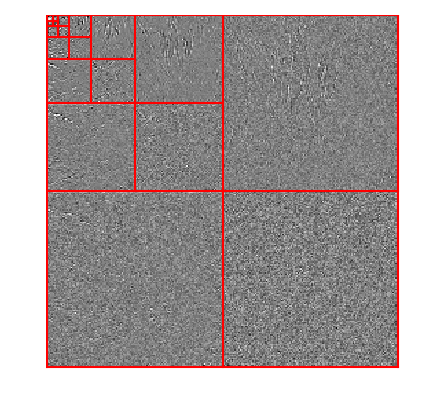Select the threshold value, that should be proportional to the noise level $\si$.

In :
T = 3*sigma;

Out:
0.24

Hard threshold the coefficients below the noise level to obtain $a_T(m)=s_T^0(a_m)$.

In :
aT = thresh_hard(a, T);

Out:
256×256 Array{Float64,2}:
23.4035    23.9434    18.6651    …  -0.0   0.0   0.0       -0.0  -0.0
13.6463    11.6067    18.1772        0.0  -0.0  -0.0       -0.0  -0.0
22.2646    25.0427    25.4722        0.0  -0.0   0.0       -0.0  -0.0
22.4718    29.6727    31.877         0.0  -0.0  -0.0        0.0  -0.0
3.22785    6.40001    7.23699       0.0  -0.0   0.0        0.0  -0.0
3.11166   -1.17377   -3.8898    …  -0.0   0.0  -0.0        0.0  -0.0
-1.20608   -3.05126    3.84766      -0.0  -0.0  -0.309199   0.0   0.0
-0.354801  -3.08171   -1.56658      -0.0  -0.0   0.0       -0.0  -0.0
3.07279    0.941851   1.33727       0.0  -0.0   0.0       -0.0   0.0
0.0       -1.93395    0.0           0.0   0.0   0.0        0.0  -0.0
-2.2519    -1.40219    2.87214   …  -0.0   0.0   0.0       -0.0  -0.0
0.718093  -0.68511   -1.51707       0.0   0.0   0.0       -0.0   0.0
0.866697  -1.2731     1.44171      -0.0  -0.0   0.0       -0.0   0.0
⋮                               ⋱                                ⋮
-0.0        0.0        0.0           0.0  -0.0   0.0        0.0  -0.0
0.0        0.0        0.0       …  -0.0  -0.0   0.0       -0.0   0.0
-0.0       -0.0        0.0           0.0   0.0  -0.0       -0.0   0.0
0.0        0.0       -0.0          -0.0   0.0   0.0       -0.0   0.0
0.0        0.0       -0.0           0.0   0.0   0.0        0.0  -0.0
0.0       -0.0       -0.0          -0.0  -0.0   0.0        0.0  -0.0
-0.0       -0.0       -0.0       …   0.0  -0.0   0.0       -0.0   0.0
0.0        0.0       -0.0           0.0  -0.0  -0.0       -0.0  -0.0
0.0        0.0        0.0          -0.0  -0.0   0.0       -0.0  -0.0
0.0        0.0        0.255558      0.0  -0.0   0.0       -0.0   0.0
0.0       -0.0       -0.0          -0.0  -0.0  -0.0       -0.0  -0.0
0.0        0.0        0.0       …   0.0  -0.0  -0.0       -0.0   0.0

Display the thresholded coefficients.

In :
plot_wavelet(aT, Jmin);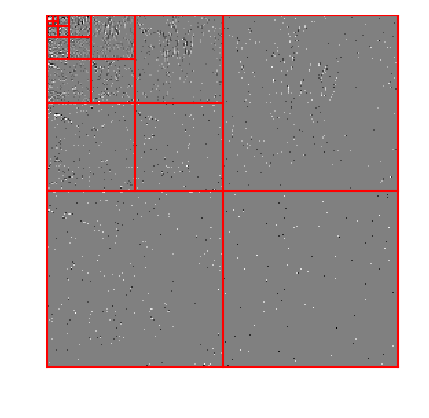Reconstruct the image $\tilde f$ from these noisy coefficients.

In :
fHard = perform_wavortho_transf(aT, Jmin, -1, h);

Out:
256×256 Array{Float64,2}:
0.162248  0.163464  0.183791  0.132795  …  0.230035  0.0966428  0.42836
0.199594  0.190882  0.198369  0.145405     0.318773  0.221399   0.386235
0.240359  0.218564  0.210504  0.151183     0.440793  0.390459   0.323647
0.268364  0.245265  0.231756  0.182457     0.438602  0.394172   0.337426
0.324586  0.294644  0.267607  0.229726     0.438543  0.42047    0.332178
0.275504  0.25939   0.24897   0.217299  …  0.43053   0.36248    0.397938
0.212396  0.216528  0.231738  0.205605     0.424056  0.271152   0.525785
0.201633  0.202059  0.209261  0.191175     0.411837  0.304244   0.421922
0.190936  0.185799  0.181536  0.17401      0.393872  0.349106   0.281461
0.179994  0.176223  0.173392  0.167054     0.397351  0.350038   0.277585
0.168208  0.164992  0.162682  0.156992  …  0.397035  0.347501   0.271225
0.159576  0.159941  0.16155   0.158516     0.41088   0.357907   0.274139
0.154099  0.161071  0.169998  0.171625     0.438887  0.381256   0.286327
⋮                                       ⋱                       ⋮
0.548284  0.538946  0.519429  0.537901     0.445084  0.463475   0.497875
0.543235  0.547109  0.547777  0.560411  …  0.450641  0.471626   0.502491
0.515679  0.545892  0.581911  0.596262     0.453934  0.478041   0.498623
0.572118  0.57968   0.59445   0.582321     0.465674  0.490937   0.526418
0.668172  0.64451   0.646581  0.552618     0.485862  0.515728   0.576498
0.61638   0.593491  0.550955  0.581742     0.474524  0.496124   0.543409
0.565821  0.543505  0.535458  0.47416   …  0.461337  0.475249   0.510077
0.510661  0.489662  0.476477  0.434131     0.455054  0.459119   0.477654
0.450898  0.431961  0.411077  0.397459     0.455675  0.447734   0.446142
0.40831   0.388657  0.369634  0.348261     0.430529  0.41864    0.411234
0.382018  0.358054  0.335697  0.307343     0.42064   0.422195   0.354509
0.29491   0.280052  0.273749  0.235521  …  0.353808  0.3039     0.379205

Display the denoising result.

In :
imageplot(clamP(fHard), string("Hard, SNR = ", string(snr(f0, fHard))) )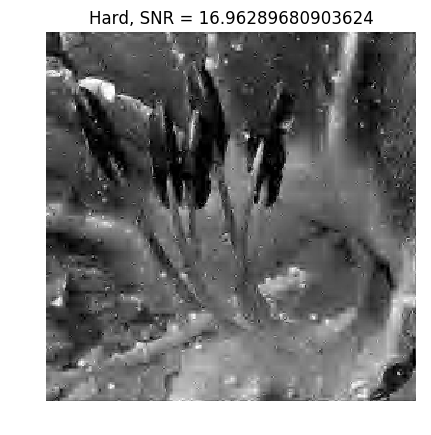Out:
PyObject <matplotlib.text.Text object at 0x0000000027583588>

## Wavelet Denoising with Soft Thesholding¶

The estimated image $\tilde f$ using hard thresholding. suffers from many artifacts. It is possible to improve the result by using soft thresholding, defined as $$\tilde f = S_T^1(f) = \sum_m s_T^1(\dotp{f}{\psi_m}) \psi_m$$ $$\qwhereq s_T^1(\alpha) = \max\pa{0, 1 - \frac{T}{\abs{\alpha}}}\alpha.$$

Display the soft thresholding function $s_T^1(\al)$.

In :
thresh_soft = (u, t) -> max(1 - t./abs(u), 0).*u
alpha = linspace(-3, 3, 1000)
plot(alpha, thresh_soft(alpha, 1))
axis("equal");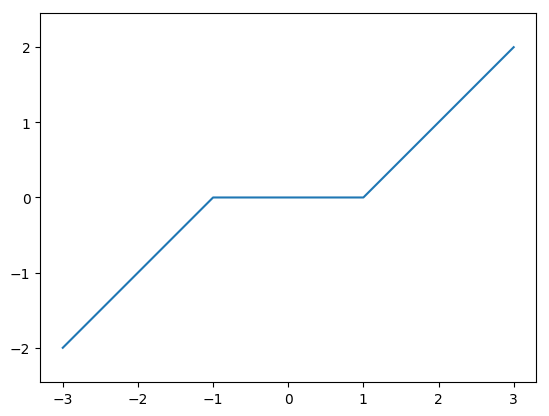Select the threshold.

In :
T = 3/2*sigma;

Out:
0.12

Perform the soft thresholding.

In :
aT = thresh_soft(a, T);

Out:
256×256 Array{Float64,2}:
23.2835      23.8234      18.5451     …  -0.0         -0.0
13.5263      11.4867      18.0572        -0.0         -0.0
22.1446      24.9227      25.3522        -0.0         -0.0
22.3518      29.5527      31.757          0.0         -0.0770398
3.10785      6.28001      7.11699        0.0282908   -0.0
2.99166     -1.05377     -3.7698     …   0.0         -0.000415364
-1.08608     -2.93126      3.72766        0.08346      0.0
-0.234801    -2.96171     -1.44658       -0.0583237   -0.0
2.95279      0.821851     1.21727       -0.0          0.0
0.0         -1.81395      0.0276036      0.0         -0.0782404
-2.1319      -1.28219      2.75214    …  -0.0         -0.116115
0.598093    -0.56511     -1.39707       -0.0          0.0471708
0.746697    -1.1531       1.32171       -0.08175      0.0607038
⋮                                    ⋱                ⋮
-0.0          0.00827876   0.0            0.0         -0.0
0.0          0.0          0.0        …  -0.0          0.0
-0.0         -0.0          0.0           -0.0          0.0
0.0          0.0         -0.0           -0.0          0.0
0.0          0.0         -0.0            0.0         -0.0
0.0         -0.0         -0.0            0.00412859  -0.0434802
-0.0         -0.0263223   -0.0        …  -0.0          0.059904
0.0          0.0         -0.0           -0.0         -0.0
0.0408282    0.0935444    0.0           -0.0         -0.0
0.0          0.0          0.135558      -0.0          0.0
0.00344442  -0.0947161   -0.0           -0.0         -0.0
0.0          0.0          0.0        …  -0.0          0.0        

To slightly improve the soft thresholding performance, we do not threshold the coefficients corresponding to coarse scale wavelets.

In :
aT[1:2^Jmin, 1:2^Jmin] = a[1:2^Jmin, 1:2^Jmin];

Out:
4×4 Array{Float64,2}:
23.4035  23.9434  18.6651  20.8802
13.6463  11.6067  18.1772  21.5199
22.2646  25.0427  25.4722  22.0171
22.4718  29.6727  31.877   31.1387

Re-construct the soft thresholding estimator $\tilde f$.

In :
fSoft = perform_wavortho_transf(aT, Jmin, -1, h);

Out:
256×256 Array{Float64,2}:
0.17342   0.172939  0.180234  0.158508  …  0.255154  0.199273  0.372073
0.220494  0.214632  0.219834  0.18375      0.367321  0.278738  0.359332
0.31405   0.223571  0.256948  0.20457      0.393764  0.382782  0.339736
0.2457    0.334712  0.228548  0.198712     0.365888  0.395095  0.345723
0.306345  0.244577  0.230353  0.213414     0.410374  0.41148   0.332919
0.262049  0.253532  0.294156  0.252261  …  0.392707  0.38587   0.374777
0.23185   0.221291  0.208176  0.204602     0.361887  0.34105   0.45209
0.216931  0.225198  0.242314  0.226402     0.380157  0.36792   0.397082
0.211362  0.208991  0.204999  0.207057     0.379989  0.343004  0.286999
0.206785  0.205693  0.202387  0.207343     0.372409  0.339608  0.283301
0.200998  0.201812  0.199364  0.20909   …  0.381969  0.345516  0.284561
0.199728  0.200107  0.197878  0.20538      0.384736  0.34371   0.282915
0.200235  0.205325  0.212106  0.212574     0.372693  0.346922  0.285712
⋮                                       ⋱                      ⋮
0.447267  0.587864  0.55065   0.481604     0.430423  0.458212  0.475337
0.482199  0.572619  0.562989  0.525241  …  0.437193  0.464077  0.485725
0.506143  0.523933  0.538793  0.57405      0.435456  0.468018  0.486883
0.571099  0.60005   0.650946  0.603556     0.465469  0.479141  0.522486
0.608928  0.602742  0.585026  0.549187     0.507303  0.479119  0.533026
0.577622  0.565062  0.527825  0.547373     0.48129   0.472961  0.515253
0.540969  0.536617  0.552423  0.493     …  0.434287  0.466616  0.496802
0.524274  0.473703  0.407297  0.399989     0.465619  0.460971  0.480883
0.467991  0.478424  0.442465  0.422222     0.434949  0.468163  0.463228
0.442474  0.381928  0.417023  0.367842     0.41544   0.430894  0.446091
0.379162  0.37951   0.384954  0.298317     0.420509  0.401609  0.429261
0.299488  0.298653  0.304555  0.243132  …  0.333849  0.342525  0.411283

Display the soft thresholding denoising result.

In :
imageplot(clamP(fSoft), string("Soft, SNR=", string(snr(f0, fSoft))) )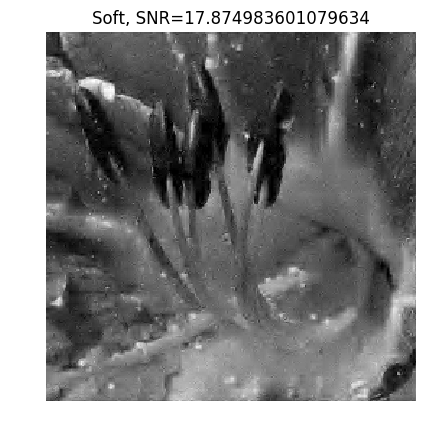Out:
PyObject <matplotlib.text.Text object at 0x00000000276D8128>

One can prove that if the non-linear approximation error $\norm{f_0-S_T(f_0)}^2$ decays fast toward zero when $T$ decreases, then the quadratic risk $\EE_w( \norm{f-S_T(f)}^2 )$ also decays fast to zero when $\si$ decays. For this result to hold, it is required to select the threshold value according to the universal threshold rule $$T = \si \sqrt{2\log(N)}.$$

Determine the best threshold $T$ for both hard and soft thresholding. Test several (T) values in $[.8\sigma, 4.5\sigma[$, and display the empirical SNR $-10\log_{10}(\norm{f_0-\tilde f}/\norm{f_0})$ What can you conclude from these results ? Test with another image.

In :
include("NtSolutions/denoisingwav_2_wavelet_2d/exo1.jl")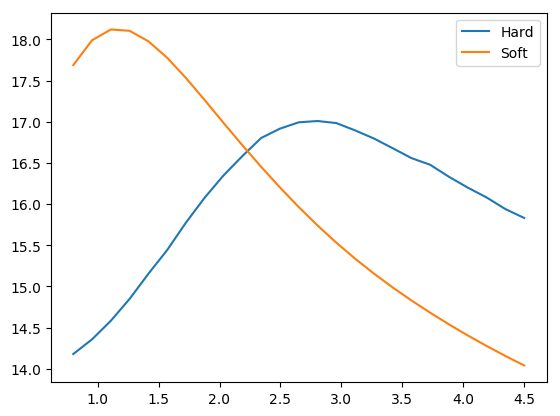Out:
PyObject <matplotlib.legend.Legend object at 0x0000000027BF7978>

## Translation Invariant Denoising with Cycle Spinning¶

Orthogonal wavelet transforms are not translation invariant. It means that the processing of an image and of a translated version of the image give different results.

Any denoiser can be turned into a translation invariant denoiser by performing a cycle spinning. The denoiser is applied to several shifted copies of the image, then the resulting denoised image are shifted back to the original position, and the results are averaged.

This corresponds to defining the estimator as $$\tilde f = \frac{1}{M} \sum_{i=1}^{M} T_{-\de_i} \circ S_T(f) \circ T_{\de_i}$$ where $S_T$ is either the hard or soft thresholding, and $T_\de(f)(x) = f(x-\de)$ is the translation operator, using periodic boundary conditions. Here $(\de_i)_i$ is a set of $M$ discrete translation. Perfect invariance is obtained by using all possible $N$ translatation, but usually a small number $M \ll N$ of translation is used to obtain approximate invariance.

Number $m$ of translations along each direction so that $M = m^2$.

In :
m = 4;

Out:
4

Generate a set of shifts $(\de_i)_i$.

In :
include("NtToolBox/src/ndgrid.jl")
(dY, dX) = meshgrid(0:m-1, 0:m-1)
delta = cat(2, reshape(dX, m*m, 1), reshape(dY, m*m, 1));

Out:
16×2 Array{Int64,2}:
0  0
1  0
2  0
3  0
0  1
1  1
2  1
3  1
0  2
1  2
2  2
3  2
0  3
1  3
2  3
3  3

To avoid storing all the translates in memory, one can perform a progressive averaging of the translates by defining $f^{(0)}=0$ and then $$\forall\, i=1,\ldots,M, \quad f^{(i)} = \pa{1-\frac{i}{n}} f^{(i-1)} + \frac{i}{n} T_{-\de_i} \circ S_T(f) \circ T_{\de_i}$$ One then has $\tilde f = f^{(M)}$ after $M$ steps.

Initialize the denoised image $f^{(0)}=0$.

In :
fTI = zeros(n, n)
T = 3*sigma
index = 1
for i in 1:m*m
fS = circshift(f, delta[i, :])
a = perform_wavortho_transf(fS, Jmin, 1, h)
aT = thresh_hard(a, T)
fS = perform_wavortho_transf(aT, Jmin, -1, h)
fS = circshift(fS, -delta[i, :])
fTI = i/(i + 1.0).*fTI + 1.0/(i + 1).*fS
index += 1
end

In :
imageplot(clamP(fTI), string("TI, SNR = ", string(snr(f0,fTI))) )